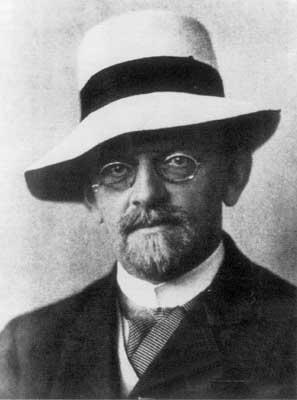# Hilbert’s ProblemsDavid Hilbert
23 Jan 1862 – 14 Feb 1943

Deciding which outstanding problems in mathematics are the most important is to decide the course of mathematics’ future development. Perhaps the mathematician who had the greatest impact on the direction of 20th century mathematics—through naming problems that most wanted attention—was the great German mathematician David Hilbert. At the second International Congress of Mathematicians, which met at Paris in 1900, Hilbert gave one of the leading addresses, in which he reviewed the basic trends of mathematical research at the end of the 19th century, and then formulated 23 problems, extending over all fields of mathematics, which he believed should occupy the attention of mathematicians in the following century.

Because of Hilbert’s prestige these problems were tackled by mathematicians assiduously, and many of them solved. Some, however, have been solved only very recently, and still others continue to daunt us. Each of these famous problems, with commentary where appropriate, is listed below.

1. The Continuum Hypothesis.

Kurt Gödel proved in 1938 that the Generalized Continuum Hypothesis (GCH) is consistent relative to Zermelo Fränkel set theory. In 1963, Paul Cohen showed that its negation is also consistent. Consequently, the axioms of mathematics as currently understood are unable to decide the GCH. See Gödel’s Theorems.

2. Whether the axioms of arithmetic are consistent.

Kurt Gödel proved in 1933 that no theory (such as a arithmetic) is strong enough to prove its own consistency. However, Zermelo Fraenkel Set Theory can prove the consistency of (the Peano axioms) of arithmetic. See Gödel’s Theorems.

3. Given any two polyhedra of equal volume, is it always possible to cut the first into finitely many polyhedral pieces which can be reassembled to yield the second?

This was proved false by Max Dehn in 1900.

4. To construct all the metrics in which straight lines are geodesics.
5. How far Lie’s conception of continuous groups of transformations is approachable without assuming that the transformations are differentiable.

It was shown in the 1950’s that every locally Euclidean group is a Lie group.

6. To axiomatize mathematical physics.

Partially solved. In particular, John-Von-Neumann and others axiomatized quantum mechanics.

7. Whether $$a^b$$ is transcendental, where $$a$$ is algebraic and $$b$$ is irrational.

This problem was solved (in the affirmative) independently by Gelfond (1934) and Schneider (1935). See the Gelfond-Schneider Theorem.

8. The Riemann (zeta) Hypothesis.

Unsolved. See the Riemann Hypothesis, zeta function.

9. To find the most general law of reciprocity in an algebraic number field.

Solved by Artin in 1927 for abelian extensions of the rational numbers, but the non-abelian case remains open.

10. To find a method to determine whether a given Diophantine Equation is soluble.

This decidability problem is kin to the larger problem pursued by the logicist program of decidability of theories in general. The particular case of Diophantine equations was finally dealt with in a direct way by Matijasevich in 1970, who showed that no such method exists.

11. The study of quadratic forms with algebraic coefficients.
12. The study of any algebraic number field extensions.
13. To show that the general equation of the seventh degree cannot be solved by means of functions of only two arguments.
14. Whether the ring $$K \bigcap k[x_1,\ldots, x_n]$$ is finitely generated over $$K$$, where $$K$$ is a field, $$k[x_1,\ldots, x_n]$$ is a polynomial ring, and $$k$$ is a subset of $$K$$, which is in turn a subset of $$k[x_1,\ldots, x_n]$$.

Proved false by Nagata in 1959.

15. The rigorous foundation of Schubert’s Enumerative Calculus.
16. The investigation of the topology of algebraic surfaces.
17. The expression of a definite rational function as a quotient of sums of squares.

Artin showed in 1927 that a positive definite rational function is a sum of squares.

18. Whether there exist non-regular space-filling polyhedra, and what is the densest sphere-packing?

The first question was answered in the affirmative by Karl Reinhardt in 1928.

19. Whether the solutions of Lagrangians are always analytic.
20. Whether every variational problem has a solution, provided suitable assumptions are made about boundary conditions.
21. To show that there always exists a linear differential equation of the Fuchsian class, with given singular points and monodromic groups.

Solved by Deligne in 1970.

22. Uniformization of analytic relations by means of automorphic functions
23. Development of the calculus of variations.